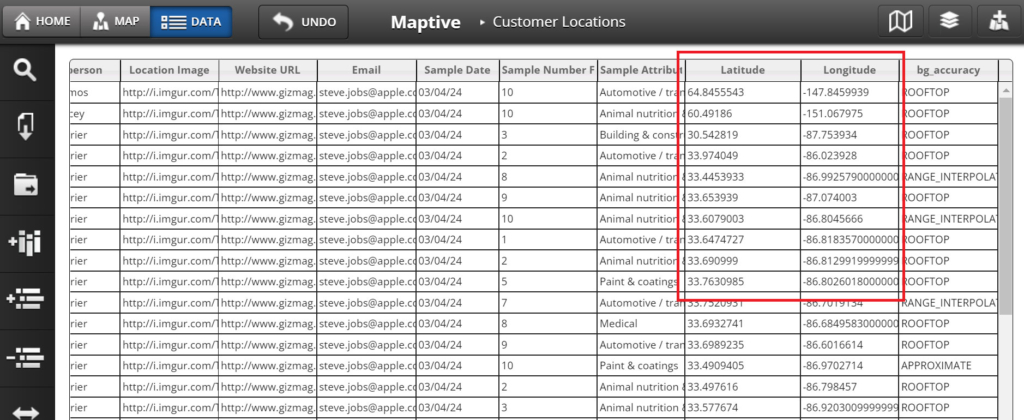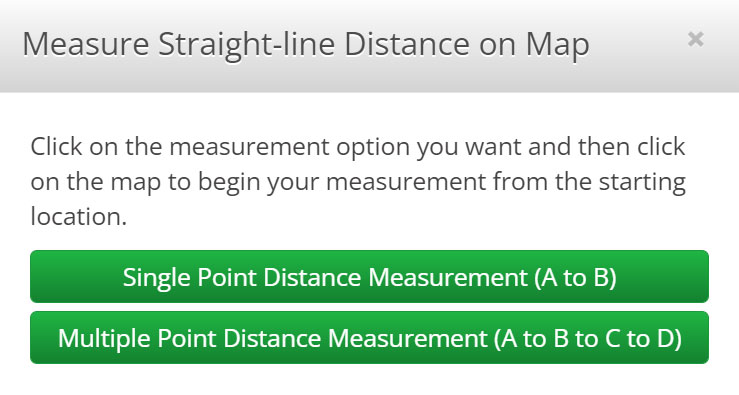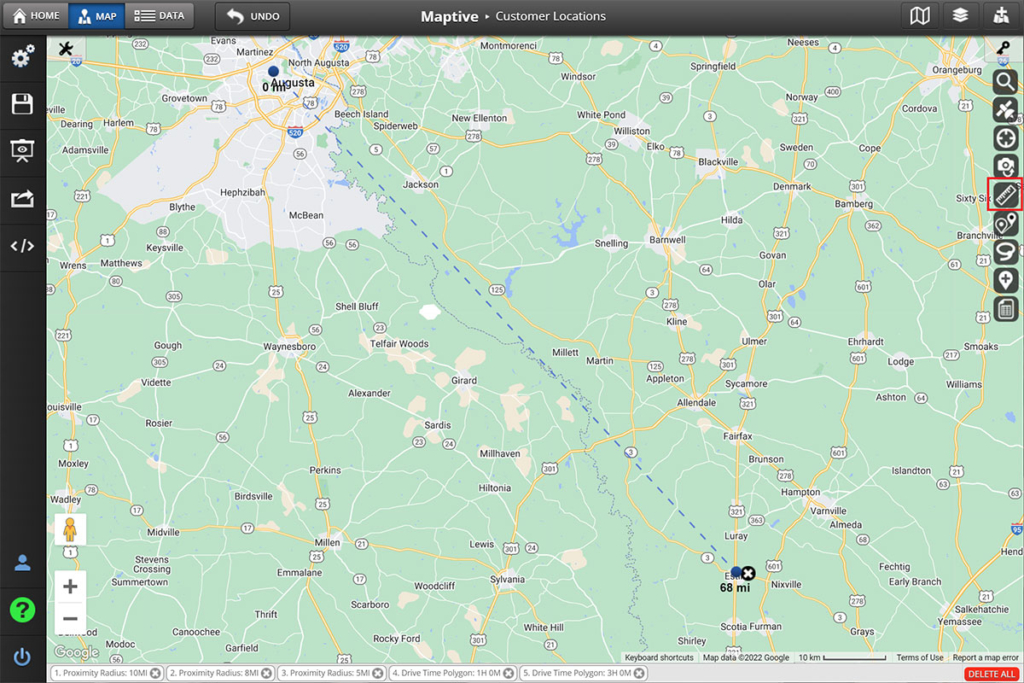# How to Measure Distance Between 2 Addresses or Points

There are a few different ways you can calculate distances between two points or addresses, and in this article, we will explore the most common ways of doing just that. There are several methods available to measure the distance between two points depending on whether you are trying to measure straight line distance or driving distance and whether you are using addresses, coordinates, city names, or other points of data.

## Main Methods of Calculating Distance

There are a variety of ways to calculate the distance between two points, or addresses.

### Straight Line Distance

The term “straight line distance” is used to describe the distance between two points without taking into account any obstructions that may be in the way. This type of distance is also sometimes referred to as a “line of sight” distance. To determine the straight line distance between two points, one would simply need to draw a line between those points on a map and then measure the length of that line. It is important to note that this method of measuring distance does not take into account any obstacles that might be present, such as mountains or bodies of water. As such, the straight line distance between two points is often significantly shorter than the actual distance that would need to be traveled in order to reach those points.

### Great Circle Distance

The great circle distance is the shortest distance between two points on a sphere. This is often the distance used when measuring the distance between two coordinates, as it is the most accurate method. The great circle distance can also be used when determining driving directions, as it gives the shortest route between two points. However, it is important to note that the great circle distance is not the same as the straight line distance. The straight line distance is the direct route between two points, regardless of whether or not there are roads that connect them. As a result, the straight line distance will often be shorter than the great circle distance. The drive time and drive distance are also different concepts. The drive time is the amount of time it would take to drive from one point to another, while the drive distance is the actual length of the journey. In general, the drive time will be longer than the drive distance, as there are often traffic delays and other factors that can add to the travel time.

### GPS Coordinates

GPS devices can also be used to calculate distance. GPS devices triangulate their position using signals from satellites and then use that information to calculate distances between two points. While GPS devices are usually quite accurate, they can be affected by factors such as interference from buildings or trees. As a result, they are not always 100% accurate.

### Latitude and Longitude Coordinates

Coordinates of locations can be plotted on a map using software like Maptive. The distance between locations can be measured using either a straight-line measurement or a route planner can be used to determine drive time or driving distances between coordinates. This can be done by inputting the coordinates or addresses of locations into a mapping program in order to map them and using a route planner to generate routes between those points.### Distance Measurement Tools

Mapping tools like Maptive or Google maps provide distance calculator tools. This allows you to click on two points on a map, showing you the straight line distance between them. All of these methods can be useful for measuring distance depending on your specific needs.

## Use Maptive to Measure Distance on a Map

Maptive is a mapping software that allows users to input location data in different formats, such as lists of addresses, coordinates, or manually added markers, and generate maps from it. The software also has a measurement tool that can be used to calculate the distance between two points. To use this tool, simply click the “Distance Calculator” button, then select “Multiple Point Distance measurement” and then click on two (or more) points you want to measure the distance between.The distance will be displayed in both miles and kilometers directly over the line you create. It is also possible to measure the area of a polygon by connecting all of the polygon’s vertices to create a closed area. Overall, Maptive’s measurement tool is a quick and easy way to get accurate measurements without using a separate GPS device. Maptive’s distance measurement tools can be very useful for businesses or individuals who need to measure the size of plots of land and distances between points of interest, such as nearby cities or potential store locations.## Using GPS Coordinates to Measure Distance with Maptive

GPS coordinates are a great way to measure distance, and Maptive makes it easy to do. Simply enter the GPS coordinates of your locations into the data tab, and Maptive will add markers on the map for each of the coordinate pairs. You can then use Maptive’s measurement tools to measure the distance between marker locations or create routes between them in order to calculate drive time or driving distances. You can also measure distances on the map by using Maptive’s freeform distance measurement tool to select starting and ending points on the map.

## How to Measure the Distance Between Two Cities

Maptive is a great tool for measuring the distance between two cities. To use Maptive, simply enter the addresses or names of the two cities into the text input boxes provided by the route planning tool. Once you have entered the addresses, Maptive will automatically calculate the distance between the two cities and display the results in miles. You can also choose to measure the distance in kilometers by selecting the “Kilometers” option from the drop-down menu.

Google Maps is another helpful tool for measuring the distance between two addresses. To use the distance measurement for driving, simply enter the addresses into the search bar and press enter. Then, click on the “Directions” icon in the upper-left corner of the map. Next, click on the “Driving” option and select “Get Directions.” The route will be displayed on the map, along with the total distance. This can be helpful when planning a road trip or trying to estimate travel time. It is important to note that the driving distance may be different than the actual mileage, as Google Maps takes into account things like traffic and construction. However, it is still useful for measuring the driving distance between two points. However, if you want a straight line distance between two points, you will need a tool like Maptive.

Want to try Maptive for free? Signup for our 100% FREE 10-day trial.

Find out why Maptive is the most powerful mapping tool on the market.